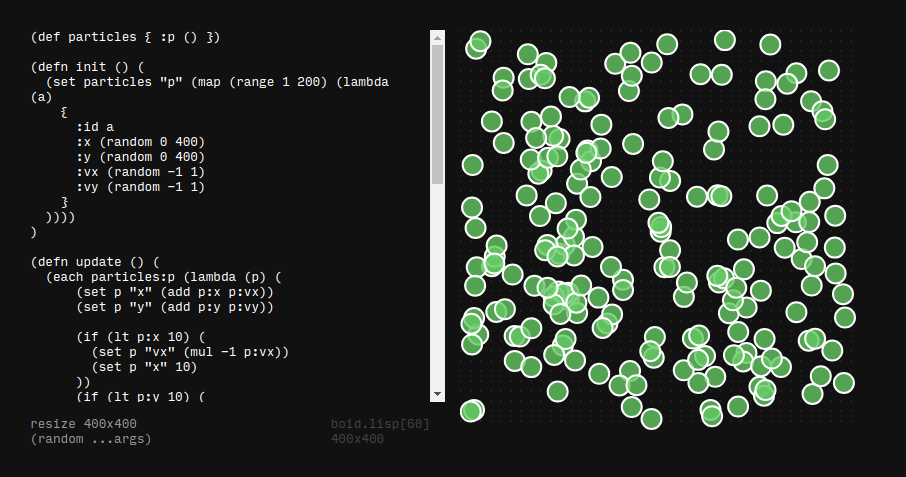## Ronin experiment 15 - particles

I have worked with particle systems a lot in processing, openframeworks and blender, so I had to experiment with one in ronin, exploring how particle states can be calculated in ronins lisp dialect.So here is the first particle system in ronin - nothing fancy like boids yet, just simple particles bouncing of the world borders.

``````(def particles { :p () })

(defn init () (
(set particles "p" (map (range 1 200) (lambda (a)
{
:id a
:x (random 0 400)
:y (random 0 400)

:vx (random -1 1)
:vy (random -1 1)

}
))))
)

(defn update () (
(each particles:p (lambda (p) (
(set p "x" (add p:x p:vx))
(set p "y" (add p:y p:vy))

(if (lt p:x 10) (
(set p "vx" (mul -1 p:vx))
(set p "x" 10)
))
(if (lt p:y 10) (
(set p "vy" (mul -1 p:vy))
(set p "y" 10)
))
(if (gt p:x 390) (
(set p "vx" (mul -1 p:vx))
(set p "x" 390)
))
(if (gt p:y 390) (
(set p "vy" (mul -1 p:vy))
(set p "y" 390)
))
)))
))
(clear)
(resize 400 400)
(init)

(defn draw (p) (
(stroke
(fill
(circle p:x p:y 10)
(rgba 100 200 100 0.8))
"white" 2)
))

(on "animate" '(
(clear)

(update)
(each particles:p (lambda (p) (
(draw p)
)))
))
``````